• 如果您觉得本站非常有看点，那么赶紧使用Ctrl+D 收藏吧

# Java8 常用的Stream流操作

2种操作：

1.intermediate  operation 中间操作：中间操作的结果是刻画、描述了一个Stream，并没有产生一个新集合，这种操作也叫做惰性求值方法。

2.terminal operation 终止操作：最终会从Stream中得到值。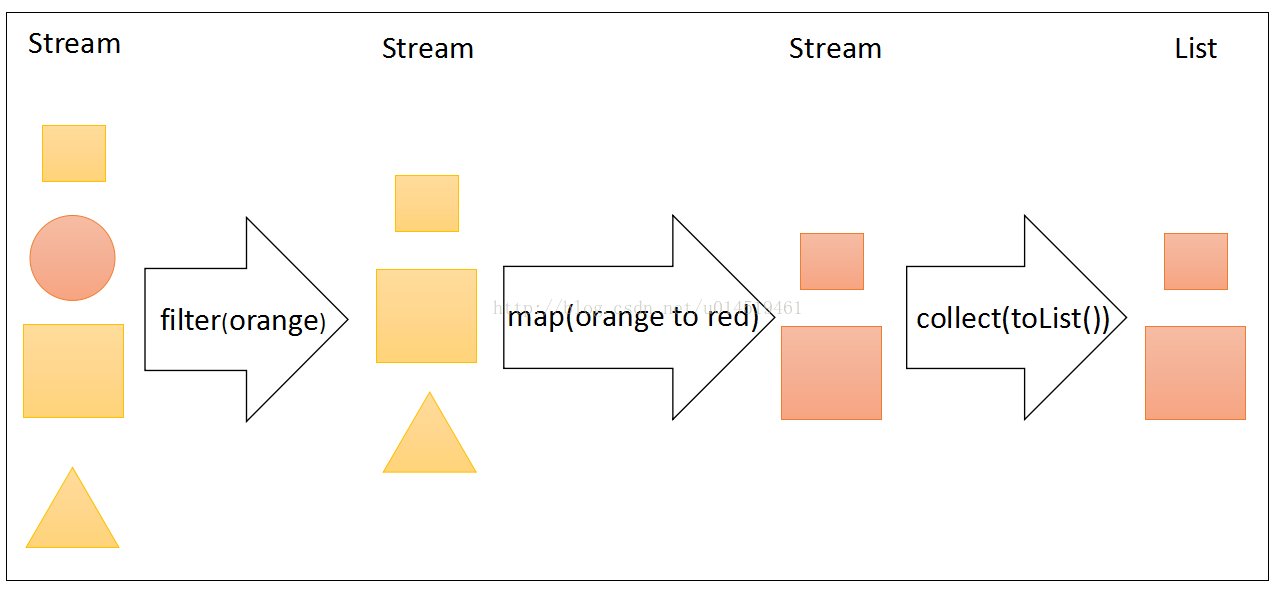1.collect(toList()) 终止操作

``````	@Test
public void collectToList() {
String[] testStrings = { "java", "react", "angular", "vue" };

List<String> list = Stream.of(testStrings).collect(Collectors.toList());

for (int i = 0, length = list.size(); i < length; i++) {
System.out.println(list.get(i));
}

}``````

2.map 中间操作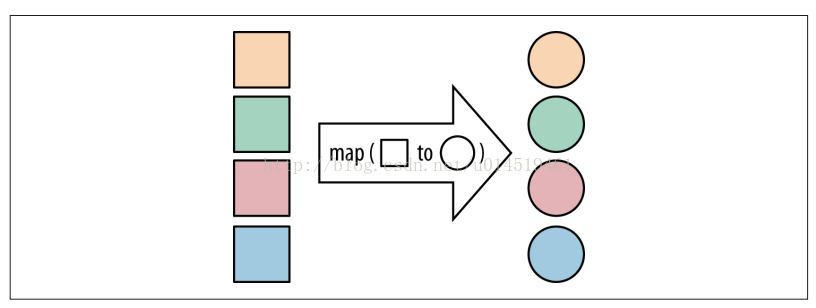``````	@Test
public void mapTest() {
String[] testStrings = { "java", "react", "angular", "vue" };

List<String> list = Stream.of(testStrings).map(test -> test.toUpperCase()).collect(Collectors.toList());

list.forEach(test -> System.out.println(test));
}``````

3.filter 中间操作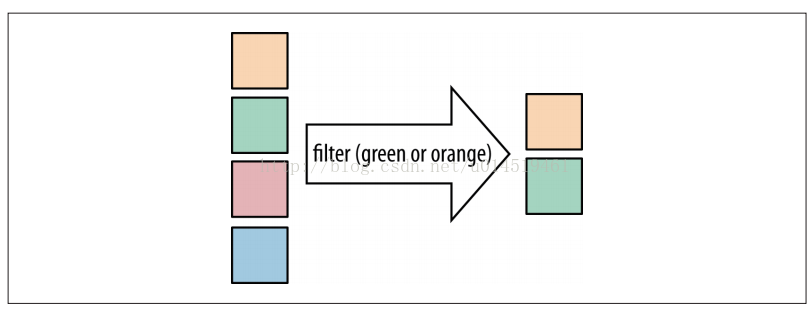``````	@Test
public void filterTest() {
List<String> list = Arrays.asList("java", "react", "angular", "javascript", "vue");

long count = list.stream().filter(p -> p.startsWith("j")).count();

System.out.println(count);
}``````

4.flatMap 中间操作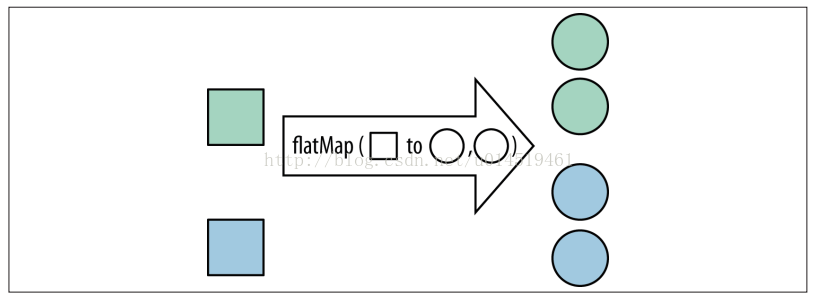``````	@Test
public void flapMapTest() {
List<Integer> list = (List<Integer>) Stream.of(Arrays.asList(1, 2, 3, 4, 5, 6), Arrays.asList(8, 9, 10, 11, 12))
.flatMap(test -> test.stream()).collect(Collectors.toList());

for (int i = 0, length = list.size(); i < length; i++) {
System.out.println(list.get(i));
}

}``````

5.max 、min 终止操作

``````	@Test
public void maxTest() {
String[] testStrings = { "java", "react", "angular", "javascript", "vue" };

Optional<String> max = Stream.of(testStrings).max((p1, p2) -> Integer.compare(p1.length(), p2.length()));

System.out.println(max);
}``````

6.reduce 终止操作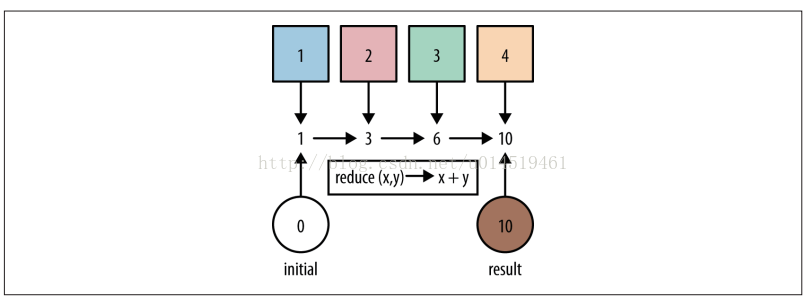``````	@Test
public void reduceSumTest() {
int sum = Stream.of(5, 6, 7, 8).reduce(0, (accumulator, element) -> accumulator + element);

System.out.println(sum);
}
``````

reduce方法的第一个参数值 0 是初始值，第二个lambda表达式参数 (accumulator, element) -> accumulator + element 是执行求和操作，其中 accumulator 是累加器，element 是每次迭代的当前元素数值。